# Mechanics Molecular Kinetic Theory Contents Mechanics n Molecular

• Slides: 25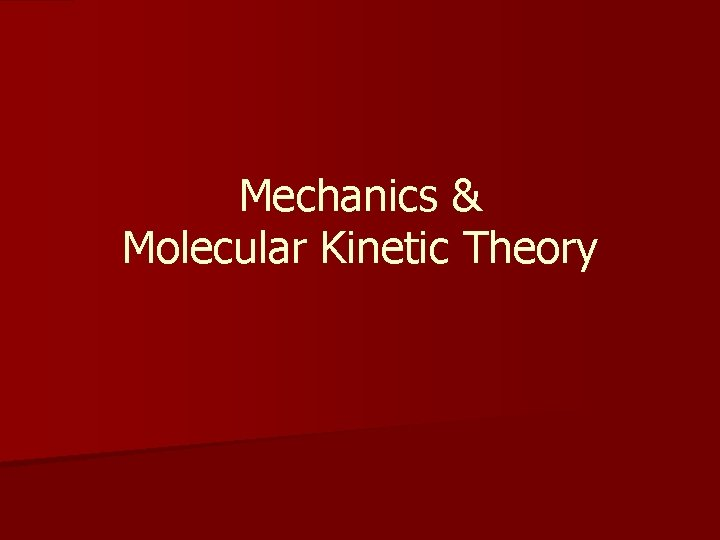Mechanics & Molecular Kinetic TheoryContents Mechanics n Molecular Kinetic Theory n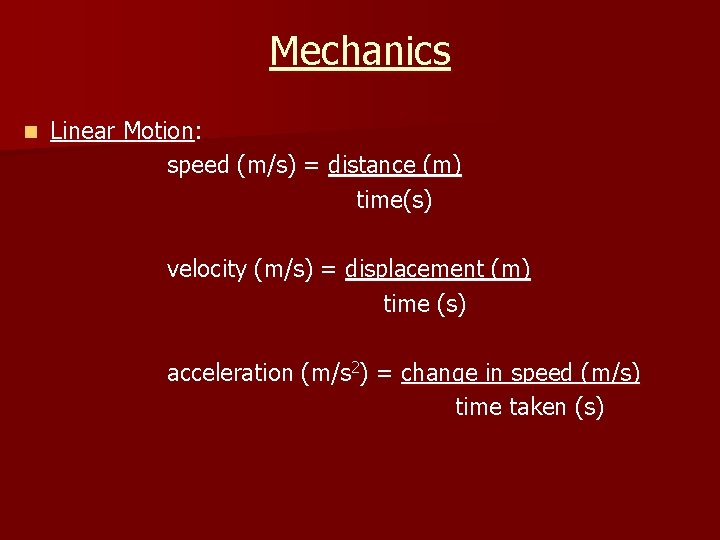Mechanics n Linear Motion: speed (m/s) = distance (m) time(s) velocity (m/s) = displacement (m) time (s) acceleration (m/s 2) = change in speed (m/s) time taken (s)Mechanics n Distance vs. Time graph: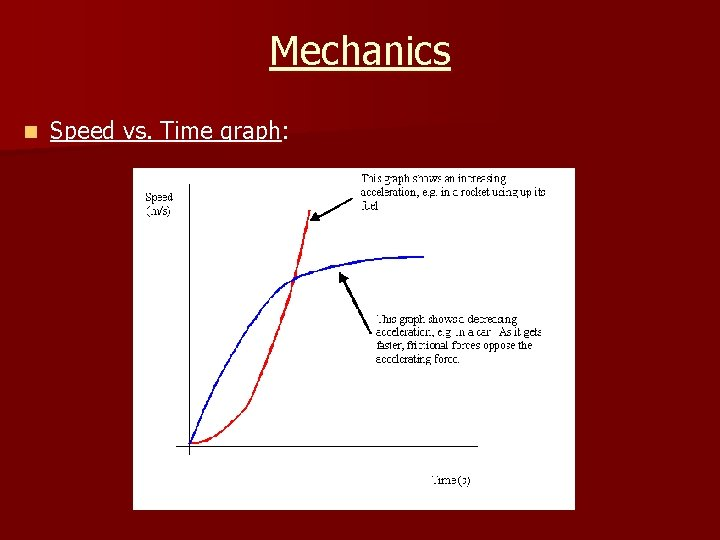Mechanics n Speed vs. Time graph: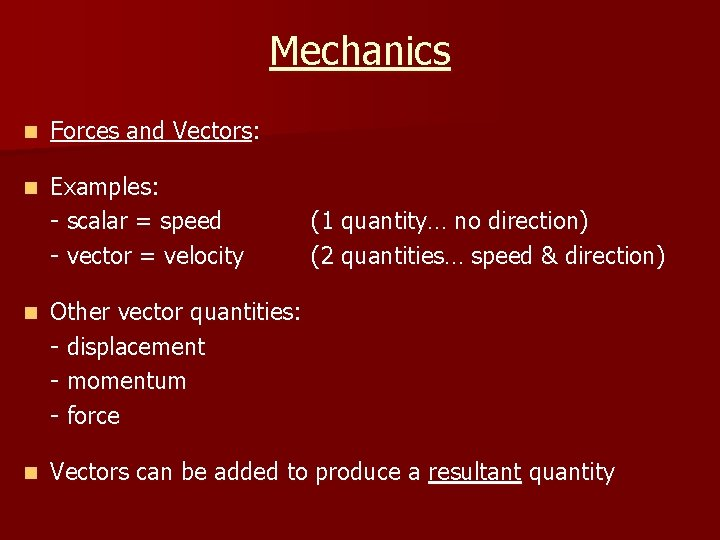Mechanics n Forces and Vectors: n Examples: - scalar = speed - vector = velocity (1 quantity… no direction) (2 quantities… speed & direction) n Other vector quantities: - displacement - momentum - force n Vectors can be added to produce a resultant quantityMechanics n Adding vectors: n And again… = + n And again… - =Mechanics n Angular mechanics: Fx = F cos Fy = F sin • Weight always faces downwards • Force on road is perpendicular to motionMechanics n Projectiles: - an object upon which the only force acting is gravity e. g. bullet - once projected, its motion depends on its inertia Initial velocity vectors: Vx = Vcos Vy = Vsin Flight time: t = Viy/g Displacement: X = Vxt Max. height: Y = Viyt + ½gt 2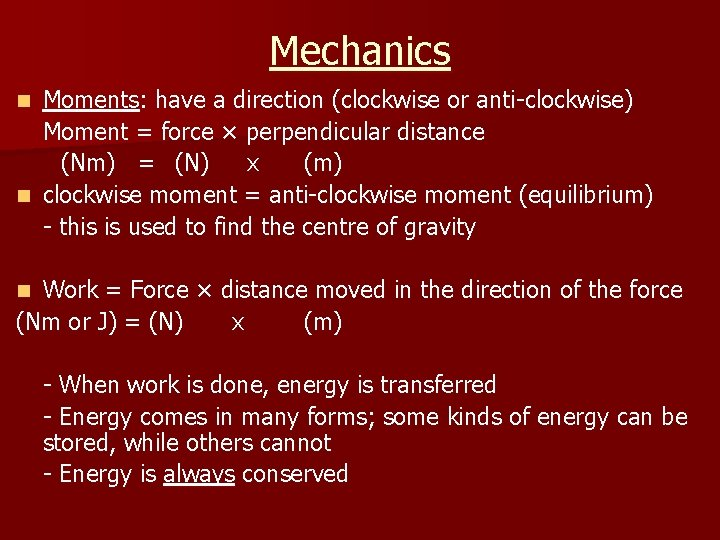Mechanics Moments: have a direction (clockwise or anti-clockwise) Moment = force × perpendicular distance (Nm) = (N) x (m) n clockwise moment = anti-clockwise moment (equilibrium) - this is used to find the centre of gravity n Work = Force × distance moved in the direction of the force (Nm or J) = (N) x (m) n - When work is done, energy is transferred - Energy comes in many forms; some kinds of energy can be stored, while others cannot - Energy is always conservedMechanics n Power: rate at which energy is transferred power (W) = energy (J) / time (secs) energy (work done) = force x distance So… power = (force x distance) / time power = force x speed P = Fv (d/t = speed)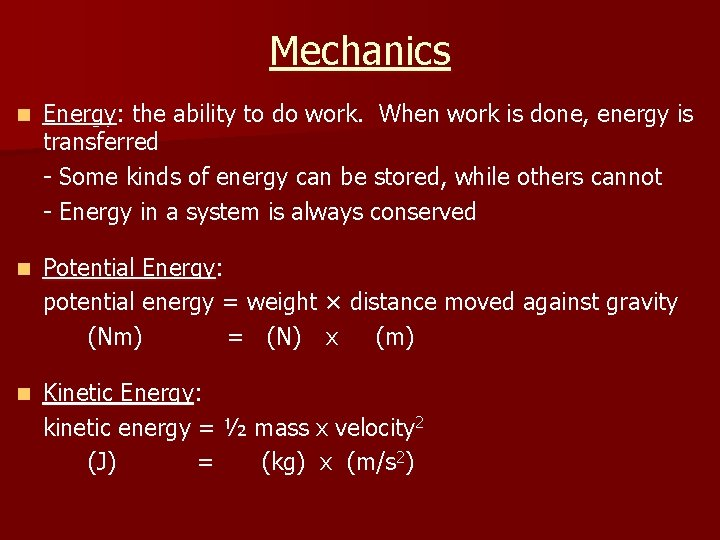Mechanics n Energy: the ability to do work. When work is done, energy is transferred - Some kinds of energy can be stored, while others cannot - Energy in a system is always conserved n Potential Energy: potential energy = weight × distance moved against gravity (Nm) = (N) x (m) n Kinetic Energy: kinetic energy = ½ mass x velocity 2 (J) = (kg) x (m/s 2)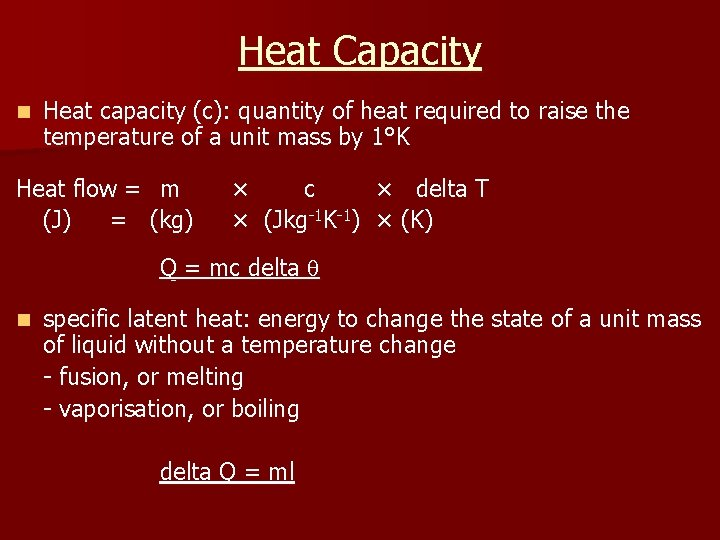Heat Capacity n Heat capacity (c): quantity of heat required to raise the temperature of a unit mass by 1°K Heat flow = m (J) = (kg) × c × delta T × (Jkg-1 K-1) × (K) Q = mc delta n specific latent heat: energy to change the state of a unit mass of liquid without a temperature change - fusion, or melting - vaporisation, or boiling delta Q = mlNewton’s Laws n Newton’s 1 st Law: An object continues in its state of rest or uniform motion in a straight line, unless it has an external force acting on it n Newton’s 2 nd Law: Rate of change of momentum is proportional to the total force acting on a body, and occurs in the direction of the force F = ma n Newton’s 3 rd Law: If body A exerts a force on body B, body B must exert an equal and opposite force on body A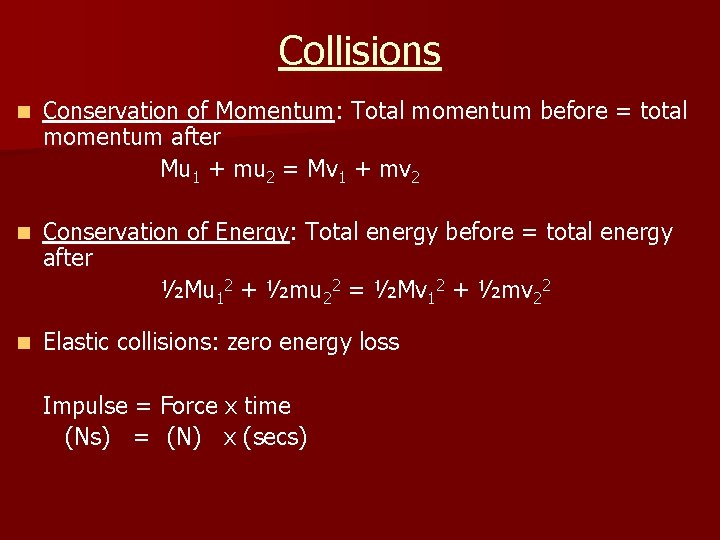Collisions n Conservation of Momentum: Total momentum before = total momentum after Mu 1 + mu 2 = Mv 1 + mv 2 n Conservation of Energy: Total energy before = total energy after ½Mu 12 + ½mu 22 = ½Mv 12 + ½mv 22 n Elastic collisions: zero energy loss Impulse = Force x time (Ns) = (N) x (secs)Ideal Gases Robert Brown investigated the movement of gas particles – 1820 s • Air particles (O 2 and N 2) – too small • Observe the motion of smoke grains Microscope Glass box Smoke grain (speck of reflected light) LightIdeal Gases Pick 1 grain & follow its movement - Jerky, erratic movement due to collisions with (the smaller) air molecules Microscope Glass box Smoke grain (speck of reflected light) LightIdeal Gases STP = standard temperature and pressure T = 273 K, p = 1 atm Average speed of air molecules = 400 ms-1 Pressure - in terms of movement of particles • Air molecule bounces around inside, colliding with the various surfaces • Each collision exerts pressure on the box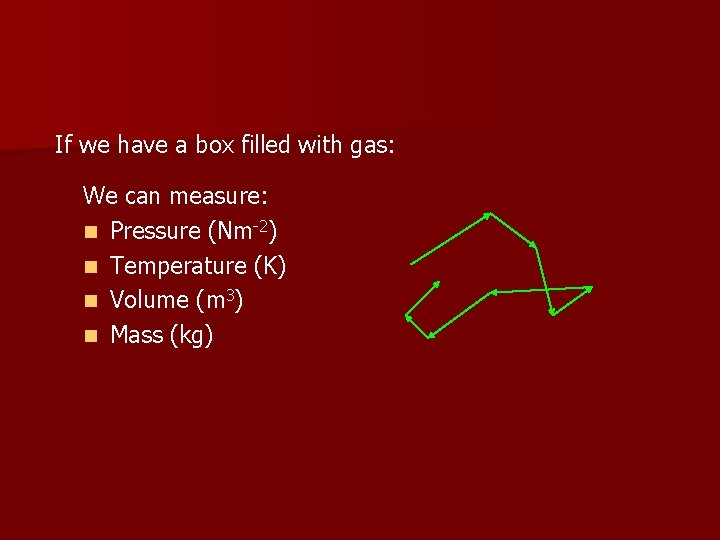If we have a box filled with gas: We can measure: n Pressure (Nm-2) n Temperature (K) n Volume (m 3) n Mass (kg)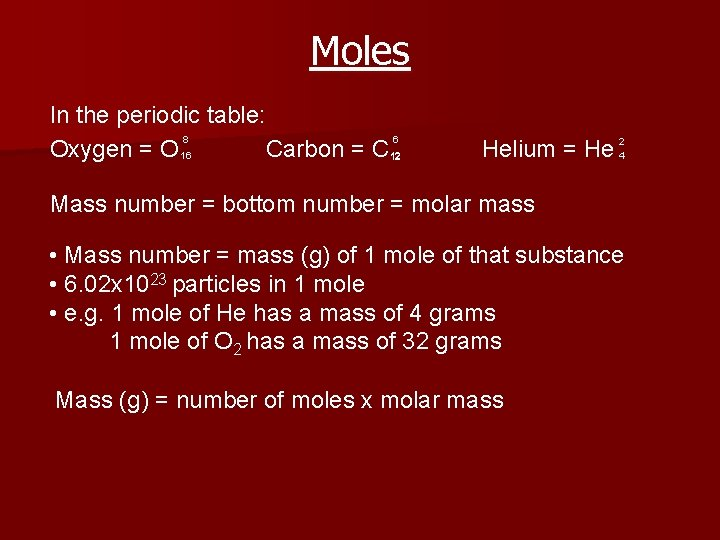Moles In the periodic table: 8 6 Oxygen = O 16 Carbon = C 12 Helium = He 24 Mass number = bottom number = molar mass • Mass number = mass (g) of 1 mole of that substance • 6. 02 x 1023 particles in 1 mole • e. g. 1 mole of He has a mass of 4 grams 1 mole of O 2 has a mass of 32 grams Mass (g) = number of moles x molar mass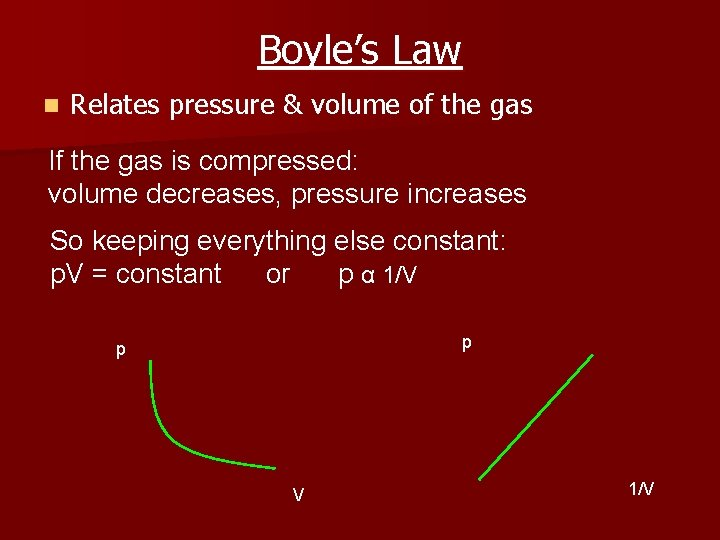Boyle’s Law n Relates pressure & volume of the gas If the gas is compressed: volume decreases, pressure increases So keeping everything else constant: p. V = constant or p α 1/V p p V 1/VCharles’ Law n Relates temperature & volume of the gas If the gas is compressed: volume decreases, temperature decreases So keeping everything else constant: V/T = constant or VαT V -300 -200 0 100 -100 200 0 100 300 T (C) 400 T (K)Pressure Law n Relates temperature & pressure of the gas If the gas is heated: temperature increases, pressure increases So keeping everything else constant: p/T = constant or pαT p 0 T (K)Ideal Gas Equation The 3 gas laws can be written as a single equation which relates the 4 properties mentioned earlier p. V = n. RT where R = universal gas constant = 8. 31 Jmol-1 K-1 n, number of moles = mass (g) / molar mass (g mol-1) e. g. how many moles are there in 1. 6 kg of oxygen? molar mass of O 2 = 32 gmol-1 number of moles, n = 1600 g/32 gmol-1 = 50 mol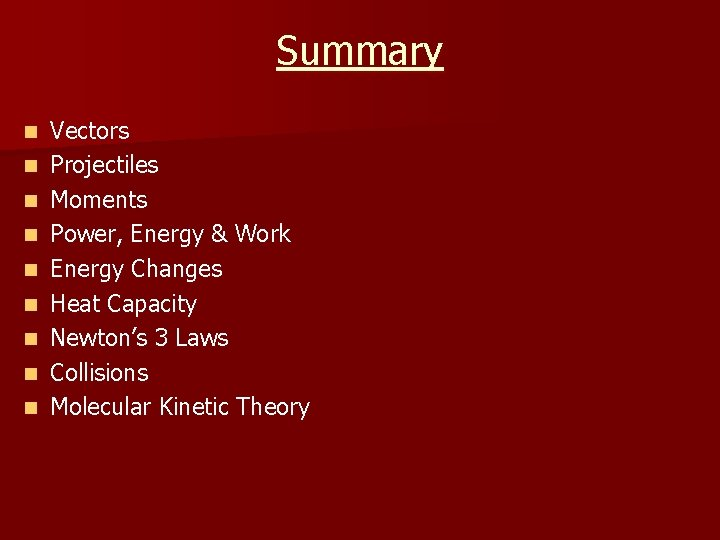Summary n n n n n Vectors Projectiles Moments Power, Energy & Work Energy Changes Heat Capacity Newton’s 3 Laws Collisions Molecular Kinetic Theory